## Problem statement

Starting in the top left corner of a 2×2 grid, and only being able to move to the right and down, there are exactly 6 routes to the bottom right corner.

## Thoughts

This could be approached recursively, as the first descision whether to go right or down can always reduce the situation to the sum of two smaller grids. The problem can be recursively reduced to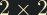and built up from there.

As I was working this out on a piece of paper though, it seemed obvious that there should be a formula for this. After a little longer than I like to admit I realised this just needs an application of the binomial coefficient! For an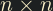grid, there'll be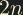total moves (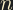right anddown). We need to choose whichare to the right, and the rest can only be down. The solution is just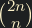.## Solution

Implement the formula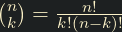and pass in 20.

Where it becomes sort of a problem is in how large the numbers get. We can keep the number "relatively low" by realising that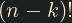is a factor of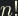, so we just need to multiply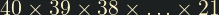and divide by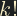, but this number is still no joke; it won't fit inside an unsigned long long, so will require bespoke handling.

We can take advantage of our knowledge that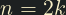to deduce that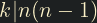, and that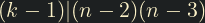. This allows us to interleave the required multiplication and division, without needing to compute two ridiculous numbers just for the sake of dividing them again.

#include <stdio.h>

int main()
{
const int num = 20;
unsigned long n = 2 * num, k = num, res = 1;

/* We only need to multiply from n down to k + 1, as the (k - 1)! is a factor of n!;
* use num as the threshold variable, as we modify k along the way. */
for (; n > num; n--) {
res *= n;
if (n % 2 == 0)
res /= k--;
}

while (k > 1)
res /= k--;

printf("%lu\n", res);
}